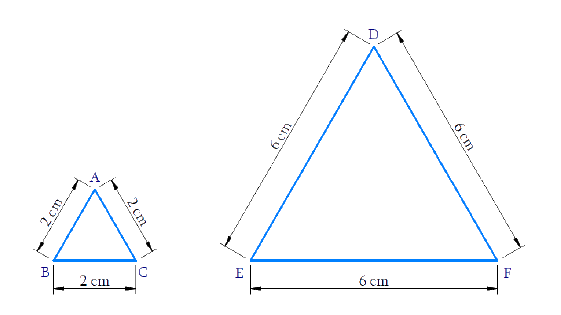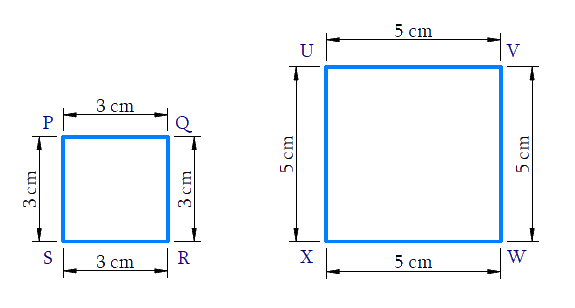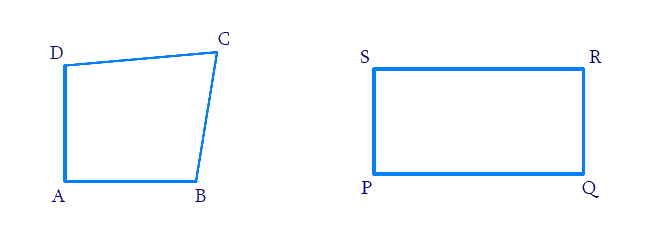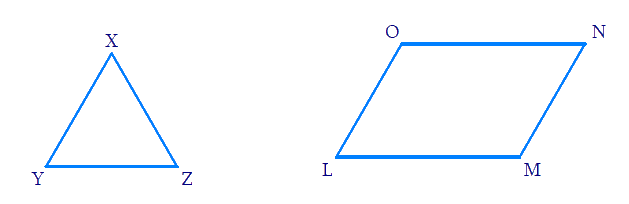# Ex.6.1 Q2 Triangles Solution - NCERT Maths Class 10

Go back to  'Ex.6.1'

## Question

Give two different examples of pair of

(i) similar figures

(ii) non-similar figures

Video Solution
Triangles
Ex 6.1 | Question 2

## Text Solution

Steps(i):

(a) Two equilateral triangles of sides $$2$$ cm and $$6$$ cm.$\Delta ABC\sim\Delta DEF\$$\sim\text{is similar to})$ (b) Two squares of sides \(3$$ cm and $$5$$ cm.$$\square PQRS\!\sim\!\square UVWX\\(\sim\text{is similar to})$$

Steps (ii) :

(a) A quadrilateral and a rectangle.(b) A triangle and a parallelogram.Learn from the best math teachers and top your exams

• Live one on one classroom and doubt clearing
• Practice worksheets in and after class for conceptual clarity
• Personalized curriculum to keep up with school